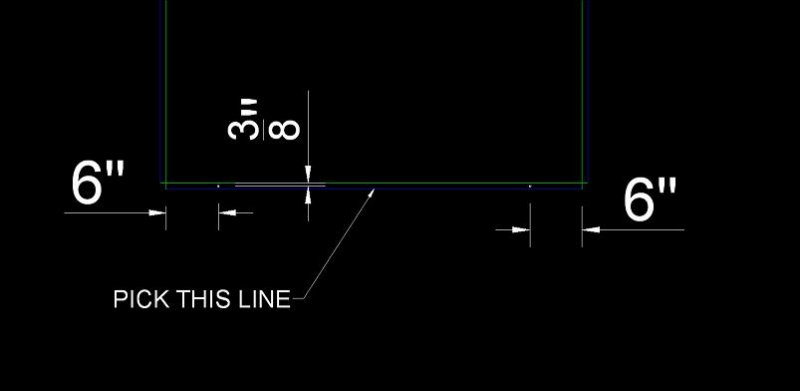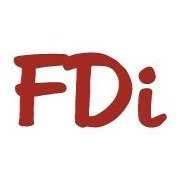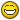# Insert Circles when line is picked

## Recommended PostsHi All,

I was wondering if there was a lisp routine out there that when you pick a line it would put to circles in between the center of two lines 6" from each end? i've enclosed a file showing what i 'm looking for.

Thanks,

Brian##### Share on other sitesDoes it mean that you want to insert a block called "holes" in a center of the selected line ?

##### Share on other sitesno. what i would like it do is draw the circles.

##### Share on other sitesThings like this .... ?

```(defun c:TesT (/ ss i sn)
(princ "\n Select lines :")
(if (setq ss (ssget '((0 . "LINE"))))
(repeat (setq i (sslength ss))
(setq sn (ssname ss (setq i (1- i))))
(entmake (list '(0 . "CIRCLE")
(cons 10
(mapcar (function (lambda (a b)
(/ (+ a b) 2.)
)
)
(cdr (assoc 10 (entget sn)))
(cdr (assoc 11 (entget sn)))
)
)
'(40 . 1.0)
)
)
)
(princ "\n Nothing selected or not line(s) *** ")
)
(princ)
)

```

##### Share on other sitesI guess this is the one .....```(defun c:TesT (/ ss i sn p1 p2)
;;; Tharwat 22. Feb. 2012 ;;;
(princ "\n Select lines :")
(if (setq ss (ssget '((0 . "LINE"))))
(repeat (setq i (sslength ss))
(setq sn (ssname ss (setq i (1- i))))
(entmake
(list
'(0 . "CIRCLE")
(cons 10
(polar (setq p1 (cdr (assoc 10 (entget sn))))
(angle p1 (setq p2 (cdr (assoc 11 (entget sn)))))
147.
)
)
'(40 . 3.0)
)
)
(entmake (list '(0 . "CIRCLE")
(cons 10 (polar p2 (angle p2 p1) 147.))
'(40 . 3.0)
)
)
)
(princ "\n Nothing selected or not line(s) *** ")
)
(princ)
)
```

##### Share on other sitesno. but thats pretty cool..i need it to put two circles 6 inch from the ends of the blue line "when picked" in my drawing and have them centered between the blue and the green lines.

##### Share on other sitesmake a couple of simple changes to code

```(polar p2 (angle p2 p1) 147.)) change the 147 to 6"
(polar p1 (angle p1 p2) 147.)) need to repeat entmake for other end

```

##### Share on other sites6" clear from both ends?

What determines the radius of the circle?

##### Share on other sitesHere is the code that Tharwat wrote and with the help of Bigal i was able to get the holes to be 6" from the end of the line like i need but what do i change to get the circles to be 3/8" above the line.

```
(defun c:wh (/ ss i sn p1 p2)
;;; Tharwat 22. Feb. 2012 ;;;
(princ "\n Select lines :")
(if (setq ss (ssget '((0 . "LINE"))))
(repeat (setq i (sslength ss))
(setq sn (ssname ss (setq i (1- i))))
(entmake
(list
'(0 . "CIRCLE")
(cons 10
(polar (setq p1 (cdr (assoc 10 (entget sn))))
(angle p1 (setq p2 (cdr (assoc 11 (entget sn)))))
6
)
)
'(40 . 0.1875)
)
)
(entmake (list '(0 . "CIRCLE")
(cons 10 (polar p2 (angle p2 p1) 6))
'(40 . 0.1875)
)
)
)
(princ "\n Nothing selected or not line(s) *** ")
)
(princ)
)
```

##### Share on other sitesI think I understand :

```[b][color=BLACK]([/color][/b]defun c:cirend [b][color=FUCHSIA]([/color][/b]/ ss en ed p10 p11 c10 c11 ang pan[b][color=FUCHSIA])[/color][/b]
[b][color=FUCHSIA]([/color][/b]and [b][color=NAVY]([/color][/b]setq ss [b][color=MAROON]([/color][/b]ssget '[b][color=GREEN]([/color][/b][b][color=BLUE]([/color][/b]0 . [color=#2f4f4f]"LINE"[/color][b][color=BLUE])[/color][/b][b][color=GREEN])[/color][/b][b][color=MAROON])[/color][/b][b][color=NAVY])[/color][/b]
[b][color=NAVY]([/color][/b]while [b][color=MAROON]([/color][/b]setq en [b][color=GREEN]([/color][/b]ssname ss 0[b][color=GREEN])[/color][/b][b][color=MAROON])[/color][/b]
[b][color=MAROON]([/color][/b]setq ed [b][color=GREEN]([/color][/b]entget en[b][color=GREEN])[/color][/b]
p10 [b][color=GREEN]([/color][/b]cdr [b][color=BLUE]([/color][/b]assoc 10 ed[b][color=BLUE])[/color][/b][b][color=GREEN])[/color][/b]
p11 [b][color=GREEN]([/color][/b]cdr [b][color=BLUE]([/color][/b]assoc 11 ed[b][color=BLUE])[/color][/b][b][color=GREEN])[/color][/b]
c10 [b][color=GREEN]([/color][/b]polar p10 [b][color=BLUE]([/color][/b]angle p10 p11[b][color=BLUE])[/color][/b] 6[b][color=GREEN])[/color][/b]
c11 [b][color=GREEN]([/color][/b]polar p11 [b][color=BLUE]([/color][/b]angle p11 p10[b][color=BLUE])[/color][/b] 6[b][color=GREEN])[/color][/b]
ang [b][color=GREEN]([/color][/b]angle p10 p11[b][color=GREEN])[/color][/b]
pan [b][color=GREEN]([/color][/b]if [b][color=BLUE]([/color][/b]< [b][color=RED]([/color][/b]* pi 0.5[b][color=RED])[/color][/b] ang [b][color=RED]([/color][/b]* pi 1.5[b][color=RED])[/color][/b][b][color=BLUE])[/color][/b]
[b][color=BLUE]([/color][/b]+ ang [b][color=RED]([/color][/b]* pi -0.5[b][color=RED])[/color][/b][b][color=BLUE])[/color][/b]
[b][color=BLUE]([/color][/b]+ ang [b][color=RED]([/color][/b]* pi  0.5[b][color=RED])[/color][/b][b][color=BLUE])[/color][/b][b][color=GREEN])[/color][/b]
c10 [b][color=GREEN]([/color][/b]polar c10 pan 0.375[b][color=GREEN])[/color][/b]
c11 [b][color=GREEN]([/color][/b]polar c11 pan 0.375[b][color=GREEN])[/color][/b][b][color=MAROON])[/color][/b]
[b][color=MAROON]([/color][/b]entmake [b][color=GREEN]([/color][/b]list [b][color=BLUE]([/color][/b]cons 0 [color=#2f4f4f]"CIRCLE"[/color][b][color=BLUE])[/color][/b][b][color=BLUE]([/color][/b]cons 10 c10[b][color=BLUE])[/color][/b][b][color=BLUE]([/color][/b]cons 40 0.1875[b][color=BLUE])[/color][/b][b][color=GREEN])[/color][/b][b][color=MAROON])[/color][/b]
[b][color=MAROON]([/color][/b]entmake [b][color=GREEN]([/color][/b]list [b][color=BLUE]([/color][/b]cons 0 [color=#2f4f4f]"CIRCLE"[/color][b][color=BLUE])[/color][/b][b][color=BLUE]([/color][/b]cons 10 c11[b][color=BLUE])[/color][/b][b][color=BLUE]([/color][/b]cons 40 0.1875[b][color=BLUE])[/color][/b][b][color=GREEN])[/color][/b][b][color=MAROON])[/color][/b]
[b][color=MAROON]([/color][/b]ssdel en ss[b][color=MAROON])[/color][/b][b][color=NAVY])[/color][/b][b][color=FUCHSIA])[/color][/b]
[b][color=FUCHSIA]([/color][/b]prin1[b][color=FUCHSIA])[/color][/b][b][color=BLACK])[/color][/b]
```

-David

##### Share on other sitesThat worked great....thanks##### Share on other sitesAnother

```(defun c:acir  (/ circle _moveblk aDoc ss e ang pts ln p1 p2 cir1 cir2 entm_ entm gr code cord)
(if (not (member "geomcal.arx" (arx)))
)
(setvar 'osmode 0)
(defun circle  (Doc p r)
(vlax-invoke
(vlax-get (vla-get-ActiveLayout Doc) 'Block)
)
)
(defun _moveblk  (flg ent bp np)
(if (not flg)
(vla-move
(setq flg (vla-copy ent))
(vlax-3d-point bp)
(vlax-3d-point np)
)
(progn (vla-delete flg) (setq flg nil))
)
flg
)
(if
(setq ss (ssget '((0 . "LINE"))))
(repeat (setq i (sslength ss))
(setq e (ssname ss (setq i (1- i))))
(redraw e 3)
(setq ang (apply 'angle
(setq pts  (mapcar
'(lambda (d)
(cdr  (assoc
d
(entget e))))
'(10 11))
)))
(if (> (setq ln (apply 'distance pts)) 12.0)
(progn
(redraw e 3)
(setq p1 (Car pts)
(setq uPt1 (polar (vlax-curve-getPointAtDist
e 6.0) (+ ang (/ pi 2.)) 0.375))
0.1875))
(setq uPt2 (polar (vlax-curve-getPointAtDist
e (- ln 6.0)) (+ ang (/ pi  2.))  0.375))
0.1875))
(prompt "\nDrag & Pick Location rebar location:")
(while
(progn
(setq gr   (grread t 15 0)
code (car gr)
)
(cond
((and (= 5 code)(> (c:cal "ang(p1,p2,cord)") 180))
(setq entm (_moveblk entm cir1 uPt1
(setq uPt1 (polar uPt1 (+ ang (* pi 1.5)) 0.75))))
(setq entm_ (_moveblk entm_ cir2 uPt2
(setq uPt2 (polar uPt2 (+ ang (* pi 1.5)) 0.75)))) T
)
((and (= 5 code)(< (c:cal "ang(p1,p2,cord)") 180))
(setq entm (_moveblk entm cir1 uPt1
(setq uPt1 (polar uPt1 (+ ang (/ pi 2.)) 0))))
(setq entm_ (_moveblk entm_  cir2  uPt2
(setq uPt2 (polar uPt2 (+ ang (/ pi 2.)) 0)))) T
)
)
)
)
(vla-delete cir1)
(vla-delete cir2)
)
)(redraw e 4)(setq entm nil entm_ nil)
)
)(princ)
(princ)
```

It will not procees the lines if the length is less than 12 units.

Edited by pBe

## Join the conversation

You can post now and register later. If you have an account, sign in now to post with your account.
Note: Your post will require moderator approval before it will be visible.×   Pasted as rich text.   Paste as plain text instead

Only 75 emoji are allowed.